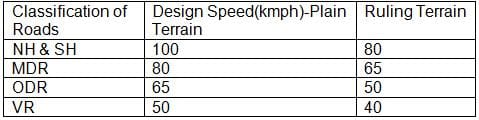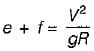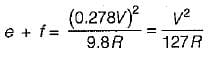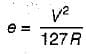# Test: Highway Geometric Design -1

## 10 Questions MCQ Test Topicwise Question Bank for Civil Engineering | Test: Highway Geometric Design -1

Description
Attempt Test: Highway Geometric Design -1 | 10 questions in 30 minutes | Mock test for Civil Engineering (CE) preparation | Free important questions MCQ to study Topicwise Question Bank for Civil Engineering for Civil Engineering (CE) Exam | Download free PDF with solutions
QUESTION: 1

### The shape of the camber, best suited for cement concrete pavements, is

Solution:

The camber is given a parabolic, elliptic or straight line shape in the cross-section. Parabolic or elliptic shape is given so that the profile is flat at the middle and steeper towards the edges, which is preferred by fast moving vehicles.
When very flat cross slope is provided as in cement concrete pavements, straight line shape of camber may be provided.

QUESTION: 2

Solution:
QUESTION: 3

### When the path travelled along the road surface is more than the circumferential movement of the wheels due to rotation, then it results in

Solution:

Skid occurs when vehicles slide without revolving or when the wheels partially revolve i.e., when the path travelled along the road surface is more than the circumferential movements of the wheels due to their rotation. Slip occurs when a wheel revolves more than the corresponding longitudinal movement along the roads.

QUESTION: 4

Coefficient of friction is less when the pavement surface is

Solution:

The coefficient of friction reduces considerably when the pavement surface is smooth or wet. The coefficient of friction also decreases slightly with increase in temperature, tyre pressure and load. But on wet pavements new tyres with good treads give higher friction factors than worn out tyres.

QUESTION: 5

Compared to a level surface, on a descending gradient the stopping sight distance is

Solution:

When there is a descending gradient the component of gravity is subtracted from the braking distance and hence the stopping sight distance is more.

QUESTION: 6

The minimum length of overtaking zone as per IRC recommendation is equal to

Solution:

The minimum length of overtaking zone should be three times the safe overtaking distance i.e., 3(d1 + d2) for one-way roads and 3(d1 + d2 + d3) for two-way roads. It is desirable that the length of overtaking zone is kept five times the overtaking sight distance.

QUESTION: 7

If the stopping distance is 60 metres, then the minimum stopping sight distance for two lane, two way traffic is

Solution:

The minimum stopping sight distance should be equal to the stopping distance in one-way traffic lanes and also in two-way traffic roads when there are two or more traffic lanes.

QUESTION: 8

The ruling design speed on a National Highway in plain terrain as per IRC recommendation is

Solution:QUESTION: 9

The equilibrium superelevation required to counteract the centrifugal force fully is given by

Solution:

As we know,e = rate of superelevation
f = lateral friction coefficient = 0.15
V = speed of vehicle, m/s
R = radius of horizontal curve, m
g = acceleration due to gravity = 9.8 m/s2
If speed of vehicle is represented as \/kmph then,If f = 0, the equilibrium superelevation required to counteract the centrifugal force fully will be given by,QUESTION: 10

The attainment of superelevation by rotation of pavement about the inner edge of the pavement

Solution:

The method of rotating about inner edge is preferable in flat terrain in high rain fall area, when the road is not taken on embankment, in order to avoid the drainage problem.Use Code STAYHOME200 and get INR 200 additional OFF Use Coupon Code